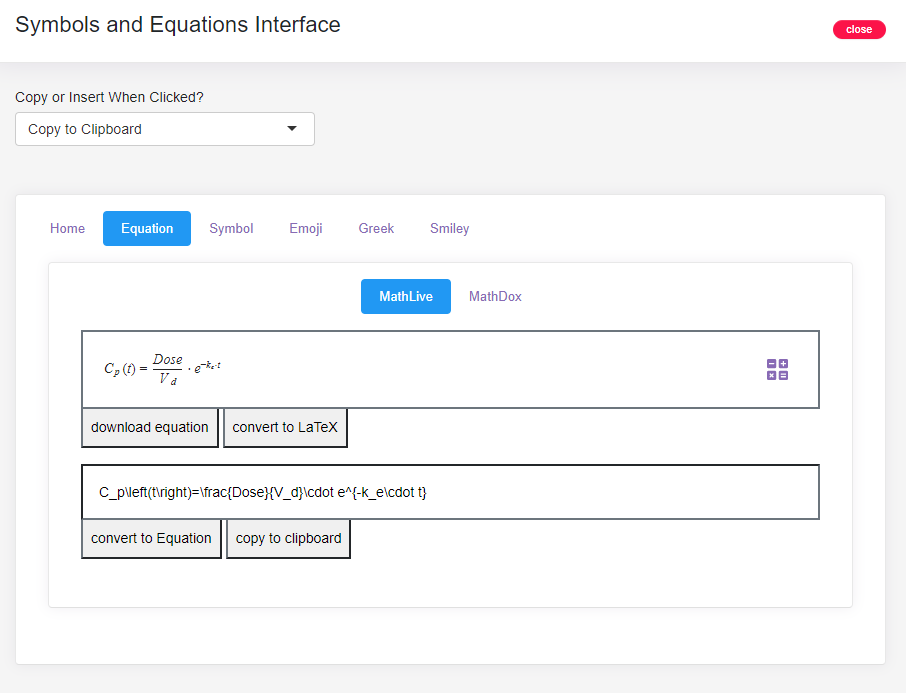# Using symbols and equations in R with the powerful symbol.equation.gpt R package

## Introduction

symbol.equation.gpt is a package that provides a user interface for building and inserting equations or symbols within R## Installation and Library Attachment

The symbol.equation.gpt package is available on CRAN and can be installed as shown below

install.packages(symbol.equation.gpt)

Attach library

library(symbol.equation.gpt)

## Use

Go to the “Addins” in RStudio and click on the “Symbols and Equations” to launch!

# Launch 2: from console

symbol.equation.ui()

# Launch 3: from console

symbol.equation.ui2()

## Examples and Demo Pages

View other packages created by me at https://coursewhiz.org